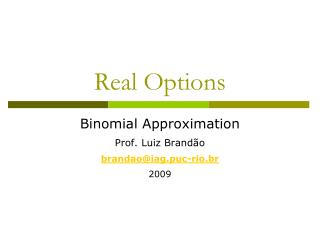DownloadDownload PresentationReal Options

# Real Options

Télécharger la présentation## Real Options

- - - - - - - - - - - - - - - - - - - - - - - - - - - E N D - - - - - - - - - - - - - - - - - - - - - - - - - - -
##### Presentation Transcript

1. Real Options Binomial Approximation Prof. Luiz Brandão brandao@iag.puc-rio.br 2009

2. Using Discrete Models • Continuous time models require relatively advanced math in order to manipulate the resulting differential equations for option value. • Nonetheless, we can model this processes more easily by dividing time in discrete periods, or time intervals. • As we adopt smaller time intervals, the values converge to the continuous time values. • This process is similar to what we usually do with the Discounted Cash Flow Methods, where continuous time cash flows are modeled as discrete monthly or annual lump sums. • In 1979, Cox, Ross e Rubinstein developed a discrete method that allows an approximation to the Geometric Brownian Motion.

3. VP4 VP3 VP2 VP1 VP0 t0 t1 t2 t3 t4 The Binomial Model • The GBM process can be approximated by a binomial lattice • This allows us to use a simpler, discrete model • The parameters for the CRR model are:

4. Example: • Using CRR, model the price evolution of an asset that follows a GBM process, assuming no dividends are paid out. • Value: \$100 million • Volatility: 15% • WACC: 12%. • No dividends. • Parameters: u, d, e probability q:

5. Example • We model initially only two periods. • Starting with the current value of the asset, we can determine possible future values and their probabilities • For this we use the CRR parameters we determined previously for the up and down movements. • Given that the process is a GBM, the distribution of values at any time t is lognormal 135,0 116,2 q 100 100 1-q 86,1 74,1

6. Exemple • Once the distribuiton of the asset prices is modeled, we can determine back its present value. • To do this, we fold back the tree starting with the payoffs of the last period, weighing these values by their risk neutral probabilities. • These values are then discounted at the risk free rate. • Note that we obtain the same value we had initially, regardless of the discount rate we use 135,0 116,2 q 100 100 1-q 86,1 74,1

7. 332,0 285,8 246,0 246,6 211,7 211,7 182,2 182,2 182,2 156,8 156,8 156,8 135,0 135,0 135,0 135,0 116,2 116,2 116,2 116,2 q 100 100 100 100 100 1-q 86,1 86,1 86,1 86,1 74,1 74,1 74,1 74,1 63,8 63,8 63,8 54,9 54,9 54,9 47,2 47,2 40,7 40,7 35,0 30,1 Exemple • We can extend this model to as many periods necessary to obtain greater precision 156,8 135,0 116,2 116,2 q 100 100 1-q 86,1 86,1 74,1 63,8

8. Real Options Binomial Approximation Prof. Luiz Brandão brandao@iag.puc-rio.br 2009